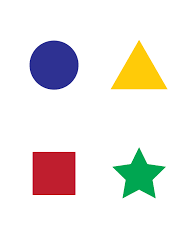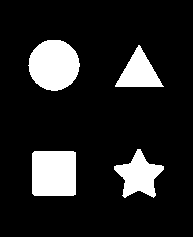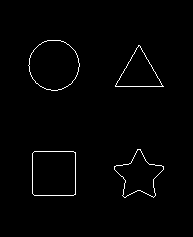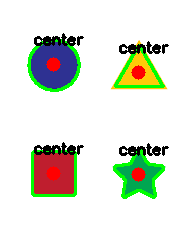GFG App
Open AppBrowser
Continue

# Python OpenCV – Find center of contour

In this article, we will learn how to find centers of contours using OpenCV in python. We will be using the findContours() and moments() functions.

We will be using the following image for center point detection:## Stepwise Implementation

Step 1: Import the required module.

## Python3

 `import` `cv2 as cv` `import` `numpy as np`

Step 2: Threshold of the image.

Before we go for contour detection, we have to threshold the above image which we can do using the following snippet:

## Python3

 `# change it with your absolute path for the image` `image ``=` `cv.imread(``"shape.png"``)` `gray ``=` `cv.cvtColor(image, cv.COLOR_BGR2GRAY)`   `blur ``=` `cv.GaussianBlur(gray, (``5``, ``5``), ` `                       ``cv.BORDER_DEFAULT)` `ret, thresh ``=` `cv.threshold(blur, ``200``, ``255``,` `                           ``cv.THRESH_BINARY_INV)`

The thresholding and the blur parameters can be changed and we would recommend you try tweaking with them to see how many contours are being detected with the given parameters.

You can save the threshold output using :

## Python3

 `cv.imwrite(``"thresh.png"``,thresh)`

Output:

`True`

This is how it should look like:We will find contours from the thresholded image using the findContours() method which returns two values, a list of all contours and their hierarchies. Now, what are contours? In simple words, a contour is a list or tree of lists of points. These points describe how a contour, that is, a vector that could be drawn as an outline around the parts of the shape based on a difference from a background.  The hierarchy on the other hand shows how the shapes relate to each other, layers as such or if shapes are on top of each other. This can be determined using hierarchies.

Step 3: Now to find the contours and the hierarchies in the thresholded image we can use the findContours() method like shown below:

## Python3

 `contours, hierarchies ``=` `cv.findContours(` `    ``thresh, cv.RETR_LIST, cv.CHAIN_APPROX_SIMPLE)`

For simplicity and to understand how contours  work and to check how many contours we can find in a thresholded image we can draw them on a blank image matrix using the following snippet:

## Python3

 `blank ``=` `np.zeros(thresh.shape[:``2``], ` `                 ``dtype``=``'uint8'``)`   `cv.drawContours(blank, contours, ``-``1``, ` `                ``(``255``, ``0``, ``0``), ``1``)`   `cv.imwrite(``"Contours.png"``, blank)`

Output:

`True`

this is how the image should look like:Step 4: File all the center points and draw them on the image.

Now for finding out the center points we will be using the moments() method. Here we will use it to find the image moment for a particular contour. An image moment is a particular weighted average of image pixel intensities, with the help of which we can find some specific properties of an image for example radius, area, centroid, etc.

To find the centroid of the image, we use the particular formula:

cx =   (M10 / M00 )

cy =  ( M01 / M00 )

where cx and cy  are the x and y coordinates of the center point and M is the moment

The following snippet finds all the center points and draws them on the image.

## Python3

 `for` `i ``in` `contours:` `    ``M ``=` `cv.moments(i)` `    ``if` `M[``'m00'``] !``=` `0``:` `        ``cx ``=` `int``(M[``'m10'``]``/``M[``'m00'``])` `        ``cy ``=` `int``(M[``'m01'``]``/``M[``'m00'``])` `        ``cv.drawContours(image, [i], ``-``1``, (``0``, ``255``, ``0``), ``2``)` `        ``cv.circle(image, (cx, cy), ``7``, (``0``, ``0``, ``255``), ``-``1``)` `        ``cv.putText(image, ``"center"``, (cx ``-` `20``, cy ``-` `20``),` `                   ``cv.FONT_HERSHEY_SIMPLEX, ``0.5``, (``0``, ``0``, ``0``), ``2``)` `    ``print``(f``"x: {cx} y: {cy}"``)`

Step 5: Finally, we can save the image using :

## Python3

 `cv.imwrite(``"image.png"``, image)`

Output:

`True`

This is what the final image would look like:My Personal Notes arrow_drop_up